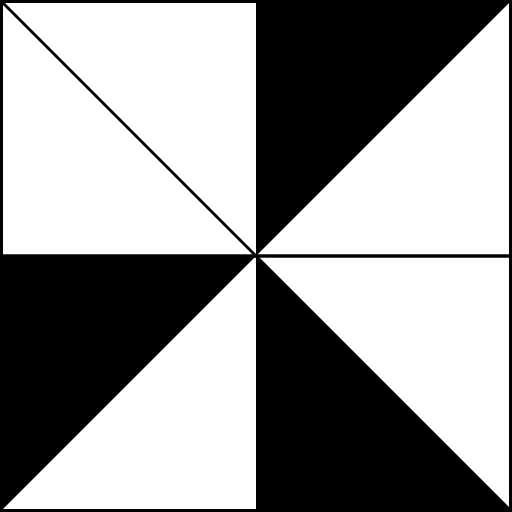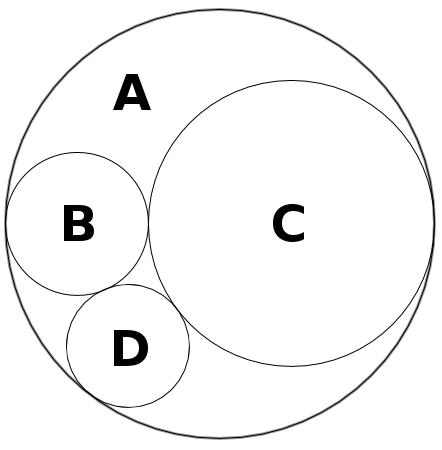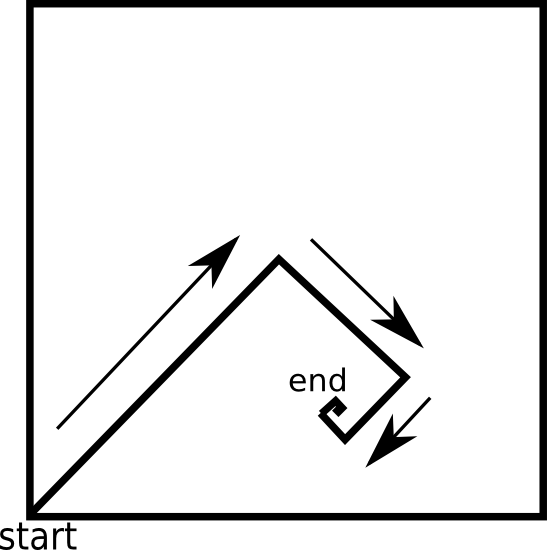Home

Puzzles

 Unfold $$a+b = 3$$ $$a^2+b^2 = 4$$ $$a^3 + b^3 = ?$$ (This problem can be solved elegantly using only algebra.) Log of a sum Consider the family of curves y = ln(e^x + e^c). -Graph the curves for various values of c using graphing software. What do you notice? -Any curve in this family can be transformed into any other curve in this family by means of a certain transformation. Describe this transformation and prove algebraically that it satisfies the previous sentence. Sum = product x,y, and c are real numbers such that x+y = xy = c. Determine the set of all possible real values for c. Lucky numbers On a lottery ticket, there are 5 distinct integers from 1 to 50 inclusive, in no particular order. Among the numbers on the ticket: The first number is the only perfect square. The second number is the only perfect cube. The third number is the only even number. The fourth number is the only prime number. The fifth number is the sum of the other four numbers. Determine the five numbers. Solution Zigzag A sequence of points is plotted on the coordinate plane: (0,1), (2,0), (0,3), (4,0), (0,5), (6,0)... (0,2n-1), (2n,0) ... (0,999), (1000,0). Line segments are drawn between consecutive points in this sequence. (The first few line segments are shown in the figure.) These line segments, along with the positive x- and y-axes, partition some of the first quadrant into triangles. "Proper" triangles are ones which contain no line segments in their interior. The proper triangles which border the x-axis are colored black, and the remaining proper triangles are colored gold. Find the ratio of the total area of the gold triangles to the total area of the black triangles.Solution Windmill Find and describe a function defined for all real numbers, whose graph in the cartesian plane has 90 degree rotational symmetry about the origin. Solution Creases A rectangular piece of paper ABCD is creased six times: Lengthwise and widthwise (A meets B, and A meets D) Meeting of corners (A meets C, and B meets D) Along the diagonal AC, and along the diagonal BD. All six creases are distinct. At the center of the paper, all the creases intersect, and the angles between each pair of adjacent creases are all equal. Determine the ratio of the paper's short side to its long side. Solution Self-sum numbers Suppose every letter represents an integer, and that certain numbers, when spelled out in english and when their letters are added together, equal the number. For instance, S + E + V + E + N = 7. Suppose this is true for the numbers five through twenty-two inclusive, and letter values are consistent across all these numbers. (Disregard the hyphen.) In all, sixteen distinct letters show up in these numbers. Find the sum of the values of all sixteen letters. Solution Exoplanet A planet orbits a binary star system. The two stars have the same orbit around the center of mass, orbit at the same speed, and remain at opposite endpoints throughout their 80 day year. (i.e., the stars take 80 days to revolve around each other, thus 40 days to switch places.) The planet's year is 360 days. How many times will the stars eclipse themselves (relative to the planet) during the planet's year?Solution Unique tiles Square tiles are divided into eight regions as seen below. Any of the eight regions may be either white or black. How many possible distinct tiles are there? Reflections are considered distinct, but rotations are not.Solution 3-ring circus Let A be a circle with radius 3. Circles B and C with radii 1 and 2 respectively are internally tangent to circle A, and externally tangent to each other. Circle D is placed externally tangent to B and C, and internally tangent to A. The radius of circle D is a rational number. Find this radius.Solution Robot range A square room is 20 meters on a side. A robot starts in one corner and travels in a straight line to the center of the room. From there, it does the following repeatedly: Turns 90 degrees clockwise, then travels half the distance it traveled previously. It repeats these steps indefinitely, but it takes a finite amount of time, and the robot stops on a point. What is the distance between the robot's final position and the farthest corner of the room from the robot?Solution Tangent logs For what exact value of $$b > 1$$ are the graphs of $$f(x) = b^x$$ and $$g(x) = \log_b x$$ tangent to each other? Solution
Home